﻿ 9. Anatella for the Expert Users > 9.2. About the integration of Javascript, R, Python inside Anatella > 9.2.3. Python Integration inside Anatella > 9.2.3.2. Coding in Python inside Anatella

# 9.2.3.2. Coding in Python inside Anatella

After clicking the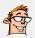icon, you switched to “Expert” mode. While in “Expert” mode, you can:

Add new parameters (and edit the parameters) of the Action: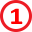See the other panels of the Python Actions: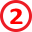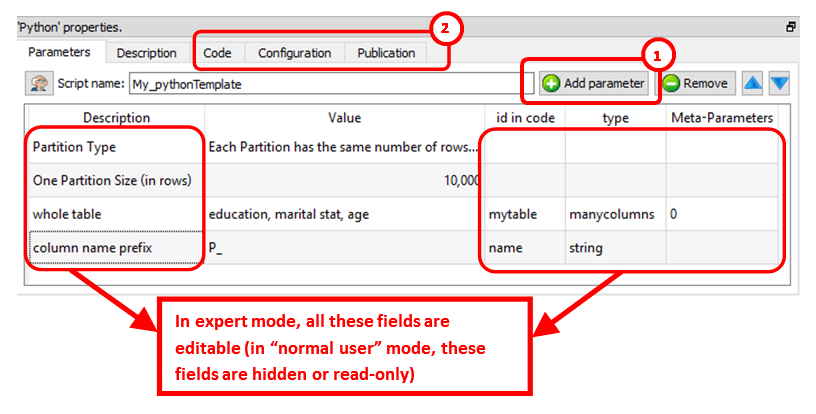In the above example, we can see that Anatella will initialize the Python engine with 2 variables (these 2 variables are named “myTable” and “name”) before running the Python code. All the parameters that are tables (coming for the input pins) are injected inside the Python engine as “Panda data frames”.

In particular, the “Code” panel is interesting: it contains the Python code: Here is an example of Python code: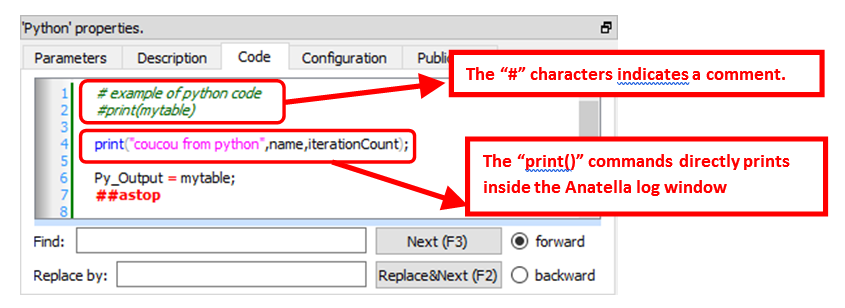You can use the "print()"command to display some values inside the Anatella Log window (this is useful to debug your code).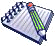When the Python engine prints some results inside the Anatella Log Window, it assumes that the font used to display the results is of Constant-Width (e.g. so that, when you print an array, the different columns from your array are correctly aligned on each row).

By default, Anatella uses a Variable-Width font inside the Log Window (and thus the array’s are not displayed very nicely).

You can change the font used inside the Log Window here: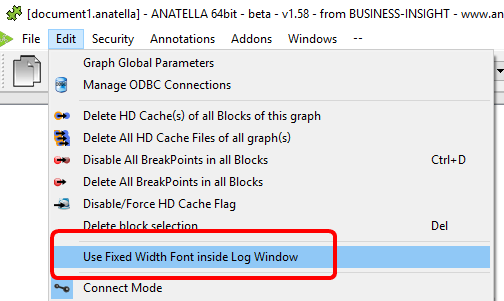(You can also use “CTRL+Wheel” to zoom in/out the text inside the Log Window)The # characters at the start of a line indicates a comment that spans over the current line only. Inside python, if you want to easily comment several lines of codes, just write ''' (three consecutive single quote characters) at the start and at the end of the block to comment (This is equivalent to /* … */ in other languages such as Javascript, C, C#, Java, etc).

Here is a special extension that is only available inside Anatella: Use the string “##astop” (without the quotes at the beginning and the end) at the very start of a line to prevent Anatella to execute any Python code located beyond the “##astop” flag. This is handy when developing new Python Actions.

Here are some “good practice” rules to follow when creating a new Python code:

1.It might be easier to use an interactive tool such as a “Jupyter Console” or “Spyder” to develop the first version of your Python code (i.e. during the first “iterations” of code development). Once your Python code is working more-or-less properly, you can fine-tune its integration inside an Anatella box using the Anatella GUI. Once the integration inside Anatella is complete, you’ll have a block of Python code (i.e. an Anatella box) that you can re-use easily everywhere with just a simple drag&drop! (…and without even looking at the Python code anymore!)When developing a new code in Python, it happens very often that the Python engine computes for a very long time (for example, because you didn’t define properly a parameter) and the whole data-transformation is “freezing” abnormally for very long period of time. In such situation, don’t hesitate to click thebutton inside the toolbar to abort all the computations prematurely. The Anatella GUI remains stable even if you cancel all the time the Python engine. This allows to make many iterations, to quickly arrive to a working code.

2.If you need a specific data-type to run your Python computations, ensure that you convert to this specific data-type before doing any computation. For example, don’t assume that you’ll always receive a matrix full of numbers in input: More precisely: Always force the conversion to the “number type”, if you specifically need “numbers” to do your computations. To convert a Pandas data frame (received as input) that is named “myDataFrame” into an NumPy Array of numbers that is named “myArray” (…and get rid of all the strings, …and keep all the column names):

myArray = df_to_array(myDataFrame);

…where we defined the function “df_to_array()” in the following way:

 def df_to_array(df):  mycols = df.columns;  mytypes=[(mycols[i], np.float64) for (i,k) in enumerate(mycols)];  return df.astype('float64').values.ravel().view(dtype=mytypes);

The function “df_to_array()” is always declared for you inside the Anatella Python engine (i.e. you don’t need to declare it yourself).

When creating a Python box that displays some plot window:

1.in the “Configuration” panel, check the option "This Action creates some plot windows" (otherwise the “plots windows” are destroyed as soon as the box stops running):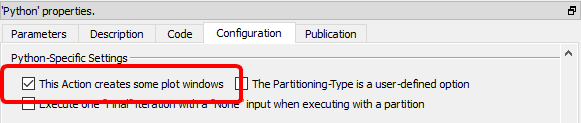2.Create the required plots, as usual, using normal python commands but DO NOT call the function “matplotlib.pyplot.show()” at the end (i.e. Anatella will do that for you later) because this function will block all code execution (i.e. the dataflow inside Anatella will be blocked as long as the plots are visible).

At the end of the execution of your Python code, the Anatella Python Engine will, in order:

a.Signal to Anatella that the execution of the python script is complete: This means that the dataflow inside Anatella may now proceed further.

b.Display all your plots by executing:

import matplotlib.pyplot as plt;

plt.show();

3.Optional: To avoid consuming much RAM memory for nothing (while Python is just busy showing your plots), add at the end of your Python code a few lines to destroy all large matrices stored in RAM. For example:

#free up memory:

myDataFrame=0; # replace the large data-frame named “myDataFrame” with

# a single number (0) to reclaim RAM

gc.collect();  # run garbage collector to force Python to release RAM

To pass back some table-results as output of the Python box, use the “Py_Output” variable. The data-type of the variable used as output is very precise: it must be a Panda data frame (and not a Numpy Array). To convert your variables to data frames, use the following command:

myDataFrame = pd.DataFrame( MyNumpyArray )

(The above command assumes that the Numpy Array already contains the correct column names: This will be the case if you used the function “df_to_array()” to create the Numpy Array).

Here are some examples of usage of the output variable named “Py_Output”:

1.To pass on output pin 0 the data frame named “myDataFrame”, simply write:

Py_Output = myDataFrame

Please ensure that the type of the variable named “myDataFrame” is indeed a “Panda data frame” (and not an “Array”), otherwise it won’t work.

2.To pass on output pin 0 the data frame named “myDataFrame1” and to pass on output pin 1 the data frame named “myDataFrame2”, simply write:

R_Output = [ myDataFrame1, myDataFrame2 ]

Please be sure to use the square brackets (“[“ and “]”) and not the parenthesis.

For the above code to work, you must also setup Anatella to have 2 output pins on your box: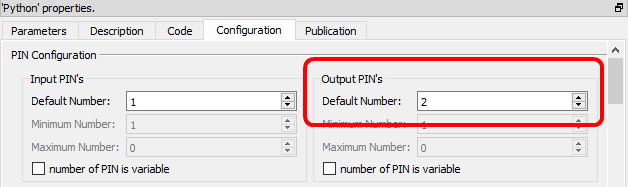To get inside the Python environment the input tables available on the second, third, fourth,... pins, use the column “Meta–Parameters” inside the “Parameters” panel:For example, this define 3 data frames (named “myDataFrame1”, “myDataFrame2” and “myDataFrame3”) that contains the tables on the input pins 0,1 & 2: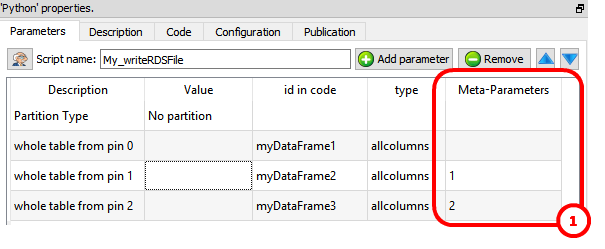The “Pandas” library (that manages Data Frames in Python) has a limitation related to the way it stores the “null” value (i.e. the “null” from the database world). More precisely, The “Pandas” library cannot store a “null” value inside the columns with the data type “int32”, “uint32”, “int64” or “uint64” (if you try to set a “null” in such a column, Pandas automatically converts the column to a ‘float64” column and thereafter set the null value). This is somewhat annoying because the columns with the “Key” meta-data-type inside Anatella are injected into python as columns with the “uint32” type. …and this Python-type does not support the “null” value (in opposition to Anatella that handles correctly the null values inside the column of the “Key” meta-data-type). To get around this limitation of python, we can choose between two options: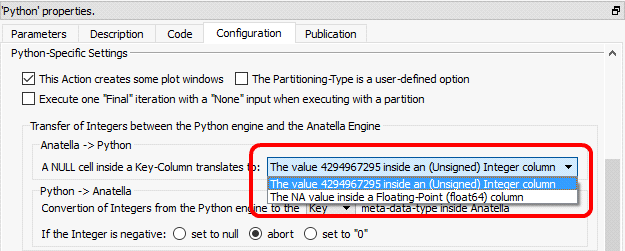These 2 options are:

1.Convert Null value to the value 4294967295 (2^32-1): This is the best & easiest option. This is perfect when the “key” columns contains “foreign keys” that are used in different joins between different tables (because the 4294967295 value will prevent the “join” to succeed, as would have done the real “null” value).

2.Use the NA value inside a column with the floating-point (“float64”) data-type: Anatella converts the column from the “Key” meta-data-type to the “Float” meta-data-type only if required (i.e. only if the column actually contained a null). This means that, when such a column “goes out” from the Python Engine back inside the Anatella Engine, its meta-data-type is undefined: it can either be “Key” or “Float” (depending if a null value was encountered). To be sure of the meta-data-type, please add a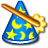ChangeDataType Action just after the Python Action (to transform back the “Float” columns into “Key” columns).

When the Anatella Python Engine starts:

It automatically creates some Pandas Data Frames containing data originating from the current Anatella Graph.

It automatically executes:

 import numpy as np;  import pandas as pd;  import gc;  import sys;

(This is required for the Pandas Data Frame to work).

This means that you don’t need to write the above “import” statements yourself.

It defines the function “df_to_array()”.

It redirects stdout&stderr to the Anatella console.

The Python engine is quite limited in terms of the size of the data it can analyze because all the data must fit into the relatively (small) RAM memory of the computer. To alleviate this limitation of Python, you can ask to Anatella to partition your data. There are currently 3 different partitioning options inside Anatella: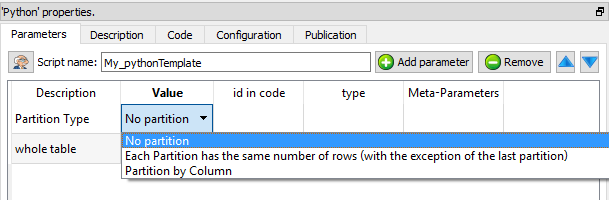How does it work? The table on the first input pin (i.e. on pin 0) is “splitted” in many different “little” tables (the tables on the other pins – pin 1, pin 2, pin 3, etc. – are always injected completely inside the Python engine without any “splitting”). The Python engine can process easily each of this “little” table because they only consume a small quantity of RAM memory. After the split, Anatella calls the Python engine “in-a-loop”, several times: At each iteration, the Python engine process one different “little” table (and it might also produce some output).

There are three “Partition Types”:

No partition (the default option): self-explaining.

Each Partition has the same number of rows (with the exception of the last partition): self-explaining.

Partition by Column: When using this option you must select a “Partitioning Column”. For example, if you select as “Partitioning Column” the column “Age”, then each partition will contain all the people (i.e. all the rows) with the same “Age”.

The concept of “Partition” is used many times inside Anatella: e.g. See the sections 5.5.3. (Partitioned Sort), 5.11.4 (Time Travel), 5.7.9. (Quantile), 5.5.9. (Flatten), 5.3.2.5. (Multithread Run), 5.11.2. (Smoothen Rows) where the same partitioning concept is also used.

More precisely, when using partitioning, Anatella does the following:

1.Split the table on pin 0 into many different “little” tables

(one table for each different partition).

2.Inject into Python the variables that contains that data from all the rows of the tables available on pin 1, pin 2, pin 3, etc.

3.Inject into Python the variable named “partitionType” (whose value is 0, 1 or 2, depending on which “Partition Type” you are using).

4.Inject into Python the variable named “iterationCount” with the value 0.

5.Inject into Python the variable named “finished” with the value “false”.

6.Run the loop (i.e. process each partition):

oInject into Python one of the “little” tables (that are coming from the “big” table available on the first input pin – input pin 0).

oRun your Python code inside the Python engine.

oGet back some output results to forward onto the output pin.

oIncrease by one the value of the Python variable “iterationCount”.

oExecute the next iteration of the loop (i.e. re-start the step 6) until there are no more “little” tables to process.

7.If the Anatella option “Execute one "Final" iteration with a NILL input when executing with a partition” is checked (i.e. it’s TRUE):

oSet the variable named “finished” to the value “true”.

oReset the variables that contains the “little” tables to NILL.

oRun the Python engine one last time.

oGet back some output results to forward onto the output pin.One example where partitioning “makes sense” is when you want to use a predictive model to score a new dataset. In opposition to “learning datasets”, “scoring datasets” can be really big (so big that they don’t fit into RAM). When you use a partition, you can compute the predictions for any (scoring) dataset, whatever the size of the dataset. Nice! J

There is also a “Kill Python” button inside the toolbar here: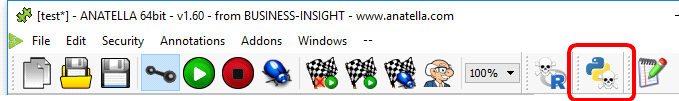This button kills all the Python engines currently running. This means that, when you click this button:

All (Python-based) plot-windows are closed (this is very handy!)

All Python computations are stopped (i.e. don’t click this button when your graph is running!).Use the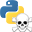button inside the toolbar to close in one click all the Python-based- plot-windows.

Once you are satisfied with your Python-based Action, you can “publish it” so that it always becomes available inside the “common” re-usable Actions: See section 9.7. for more details.